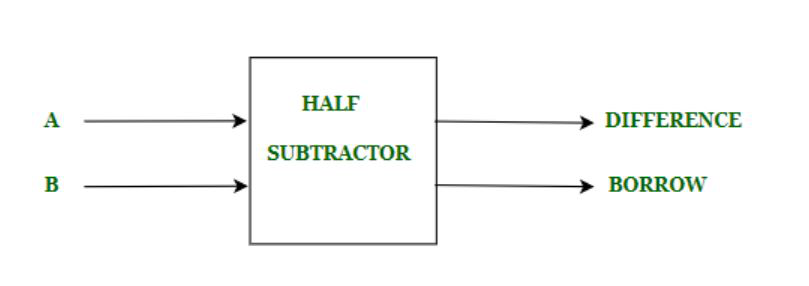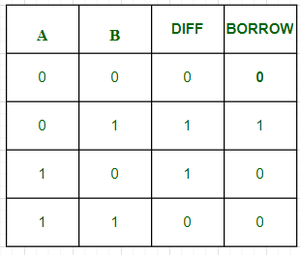Related Articles

# C++ program to implement Half Subtractor

• Last Updated : 14 Sep, 2021

Prerequisite :  Half Subtractor
We are given with two inputs A and B. Our task is to implement Half Subtractor circuit and print the outputs difference and borrow of two inputs.

Introduction :
The half subtractor is used to subtract two 1-bit numbers  which are minuend, and subtrahend . The half subtractor has two input states and two output states. The two outputs are difference and borrow. The Difference is the difference between the inputs it is set when both the inputs are different  and borrow is set when subtrahend  is greater than minuend we borrow “1” and set borrow “1”.  The block diagram of half subtractor is

Attention reader! Don’t stop learning now. Get hold of all the important CS Theory concepts for SDE interviews with the CS Theory Course at a student-friendly price and become industry ready.Here we have two inputs A, B and two outputs Difference, Borrow. The values of Difference and borrow will depend on A and B . The truth table for Half Subtractor isThe Difference is calculated by XOR operation of A and B and the borrow is calculated by And operation of Ā and B. Here Ā is NOT operation of A which gives inverse output.

Logical Expression :

```Difference = A XOR B
Borrow =     Ā AND B```

Examples –

```Input :          A=1, B= 0
Output:       Difference=1, Borrow=0
Explanation : Here from logical expression Difference = A XOR B
i.e  1 XOR 0 = 1, and Ā = NOT(A) i,e NOT(1) = 0.
So, Borrow=Ā AND B
i.e 0 AND 0 = 0.```
```Input :       A=0, B= 1
Output:       Difference=1, Borrow=1
Explanation:  Here from  logical expression Difference=A XOR B
i.e. 0 XOR 1 =1 and Ā = NOT(A) i,e NOT(0) = 1.
So, Borrow=Ā AND B
i.e 1 AND 1 =  1.```

Approach :

• Initialize the variables Difference and Borrow for storing outputs.
• First we will take two inputs A ,B.
• By applying  A XOR B  we get the value of Difference.
• By applying  NOT A   we get the value of  Ā .
• By applying Ā AND B  we get the value of Borrow.

## C++

 `// C++ program to implement Half subtractor` `#include ``using` `namespace` `std;` ` ``// Function to print Difference and Borrow``void` `Half_Subtractor(``int` `A,``int` `B){``    ``// initializing Difference and Borrow``    ``int` `Difference,Borrow;``    ``// Calculating value of Difference``    ``Difference = A ^ B;`` ` `    ``// Calculating value of Borrow``    ``// Calculating not of A``    ``A = not(A);``    ``Borrow = A & B;`` ` `    ``// printing the values``    ``cout<<``"Difference = "``<

Output :

```Difference = 1
Borrow = 0 ```

My Personal Notes arrow_drop_up